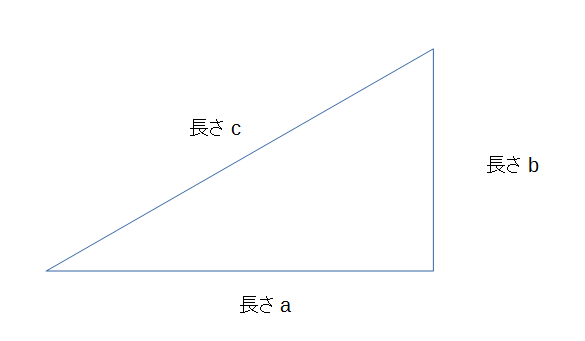# Pythagorean Theorem

Pythagorean Theorem is a theorem on the right triangle.

Suppose a right triangle, its sides a, b, and c, where c is the longest (hypotenuse).
c² = a² + b²In order to calculate the side, you need to calculate the root, which is often laborious. Here is a calculator form for Pythagorean Theorem.

### Calculting the hypotenuse

hypotenuse:

Squareroot often results in an irrational number. In the form above, the result is rounded.

### Calculating the leg

the remaining leg:

Here, the longer of side1 and side 2 will be regarded as the hypotenuse

Updated:

Tags:   math